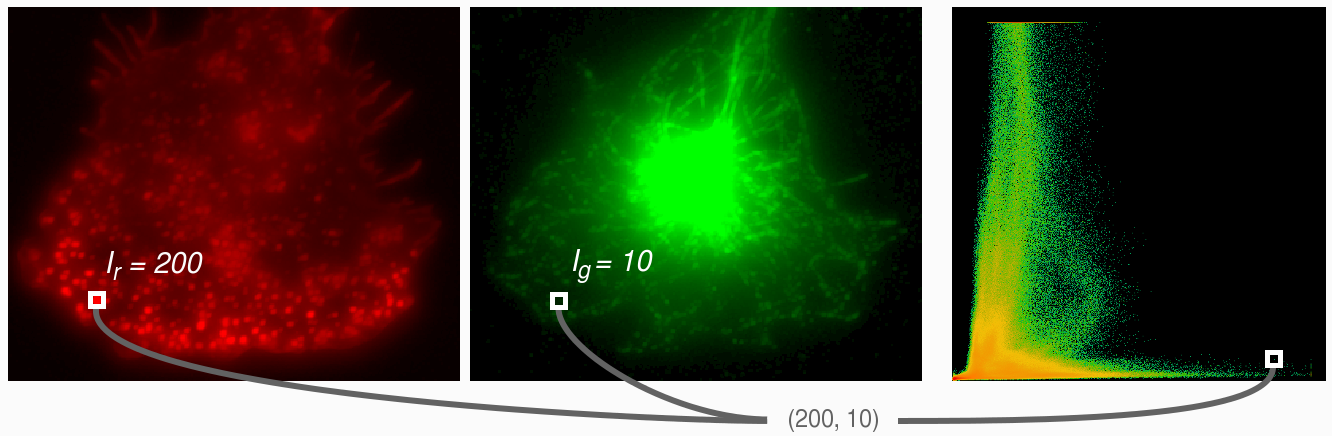# 2D histograms

Multidimensional histograms are the extension of the concept of Image Histogram to Multi Channel images. In image processing a 2D histogram shows the relationship of intensities at the exact position between two images. The 2D histogram is mostly used to compare 2 channels in a multi-channel images, where the x-axis represent the intensities of the first channel and the y-axis the intensities of the second channel.

As a comparison, a 1D histogram is nothing more than counting how many voxels with a particular intensity occur in the image. The intensity range of the image is divided in bins. A voxel then belongs to the bin if its intensity is included within the range the bin represents.

The 2D histogram is the same as the 1D histogram with the difference that it counts the occurence of combinations of intensities. To compute a 2D histogram the images need to be equal in size. See the example in the image below; at position (30,20) the first channel has an intensity of 200 and the second image has an intensity of 10. Then this will add one to the count in the 2D histogram at position (200,10).Example how a 2D histogram is computed, showing the first and second channel and their corresponding 2D histogram. At each position within the channels, the corresponding intensities of both channels are combined to form a coordinate within the 2D histogram. The count at this coordinate of the 2D histogram is then increased by one.

The difficulty with a 2D histogram is how to show the actual count per intensity combination. For a 1D histogram the height of the bars represent the count, but this height for a 2D histogram requires a third dimension which is difficult to visualize. Instead, to visualize the count of the combinations, colors are used. In the histogram in the image above colors range from green to red. Note that these colors have nothing to do with the colors used to represent the channels. Within the 2D histogram, green represents low counts while red represent high counts.

2D histograms show interesting image properties and are therefore very useful:

 Black level Within a 2D histogram the origin generally represent the (0,0). If there is a blacklevel, a.k.a. offset, in the image then the histogram contents is shifted from the (0,0) Intensity proportionIf the histogram is elongated in the x-direction, this shows that the first channel has a larger dynamic range then the second channel. CorrelationThe Colocalization Analyzer shows a 2D histogram, because the shape of the histogram tells you what kind of correlation can be expected. For two channels with a high degree of overlapping, the histogram pixels tend to concrentrate along the y=x line. The example on the left, show two equal channels with poisson noise added. In case of a perfect overlap, the line would be exactly y=x.Also anti-correlation is visualized within the histogram. The more the histogram pixels concentrate at the axis of the histogram, the more anti-correlation can be expected. ClippingClipping is shown very clearly within a 2D histogram. In the example in the left, there is clipping in the second channel. Shown by the "boundary" at the top. It is always recommended to avoid clipping, since this is unrestorable information loss which will affect deconvolution. CrosstalkWhen, for example, the first channel bleeds through the second channel (which is known as crosstalk or bleedthrough), then there is always a little signal in the second channel at the positions when there is signal in the first channel. This is visualized by an empty triangular shape, from the origin along the axis of the first channel. Hot pixels Hot pixels can be viewed within a 2D histogram, if the hotpixels are conserved between channels. In this example, the hot pixels vary in intensity, but their positions are equal in each channel. This results in the diagonal scatter. The Hot & Cold Pixel Remover can remove these hot pixels and the 2D histogram of the image after hot pixel removal shows the absence of this diagonal scatter

Keywords: two channel histogram multidimensional intensity